# Accelaration & forces in different inertial referance frames

arpon
Are the accelaration and forces in different inertial referance frame equal ?

Think about two observers moving at 1m/s less than c with respect to one another. What happens if one fires rockets to accelerate at 1g?

Staff Emeritus
Are the accelaration and forces in different inertial referance frame equal ?

No.

The post by Ibex suggests why, though when you factor in all the different relativistic effects, it's probably too hard to figure out all the details on one's own.

I'm not sure where the best reference is, Wiki has some discussion of the issues at http://en.wikipedia.org/wiki/Mass_in_special_relativity#Transverse_and_longitudinal_mass

The precise relativistic expression (which is equivalent to Lorentz's) relating force and acceleration for a particle with non-zero rest mass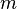moving in the x direction with velocity v and associated Lorentz factor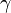is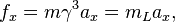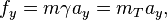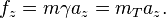•arpon
Staff Emeritus
Are the accelaration and forces in different inertial referance frame equal ?

I think your question has two different answers, depending on how you define "acceleration" and "force", and depending on what you mean by two vector quantities being equal.

Here are two different ways to define the acceleration of an object:
1. Coordinate acceleration.
2. "Proper" acceleration (which basically is acceleration relative to objects in freefall)
These are vector quantities, not numbers. There are two different ways to define equality of vector quantities:
1. Component-wise equality.
2. Covariant equality.
Let me illustrate the difference with something a little more mundane: Ordinary vectors in space. Suppose you are standing at an intersection, and there is an arrow pointing in some direction bearing the words "This way to Joe's restaurant". You might describe this arrow as pointing to the left. Then if you circle around the arrow, you might change your mind, and say that it is now pointing to right. So did the arrow change directions when you moved around? I would say no; your description of the arrow changed, because your notion of "left" and "right" changed, but the arrow didn't change.

The changes that happen to vectors (actually, 4-vectors, because in Special Relativity, there are 4 components to vectors; besides the usual 3 spatial components, there is also a time component) when you change reference frames can be thought of in the same terms. You can think of it as the vector as remaining constant, and only your description of the vector changes.

Coordinate acceleration definitely changes when you change reference frames, no matter how you slice it. Proper acceleration as a 4-vector can be thought of as remaining the same in all inertial reference frames, and the only thing that changes is the description of the 4-vector in terms of components.

•arpon
Staff Emeritus